# Section 2 Velocity and Momentum An objects velocity

• Slides: 12
Download presentation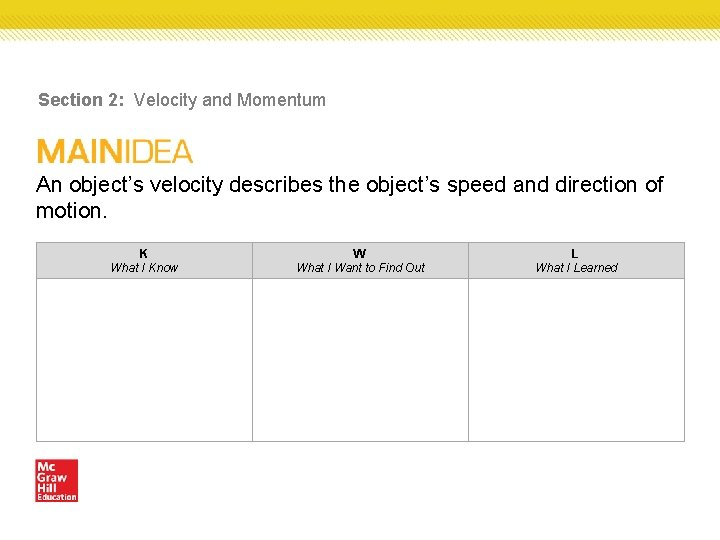Section 2: Velocity and Momentum An object’s velocity describes the object’s speed and direction of motion. K What I Know W What I Want to Find Out L What I Learned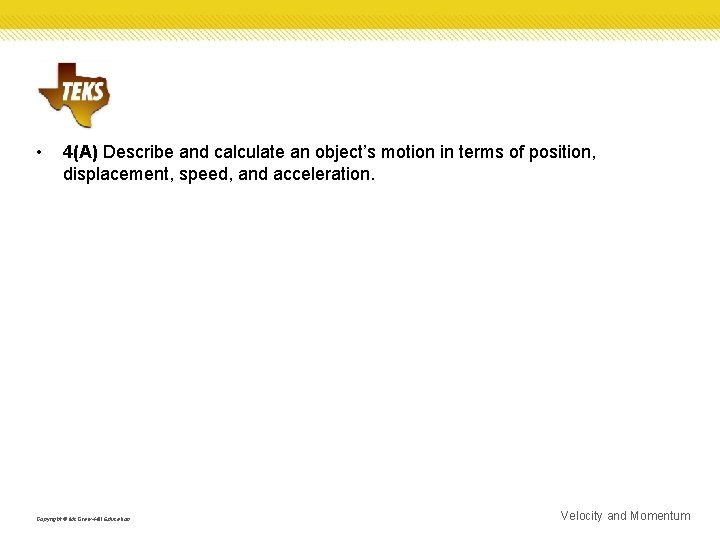• 4(A) Describe and calculate an object’s motion in terms of position, displacement, speed, and acceleration. Copyright © Mc. Graw-Hill Education Velocity and MomentumEssential Questions • What is the difference between speed and velocity? • How is the motion of two objects relative to each other described? • How can an object’s momentum be calculated? Copyright © Mc. Graw-Hill Education Velocity and MomentumVocabulary Review New • • • speed Copyright © Mc. Graw-Hill Education velocity momentum Velocity and Momentum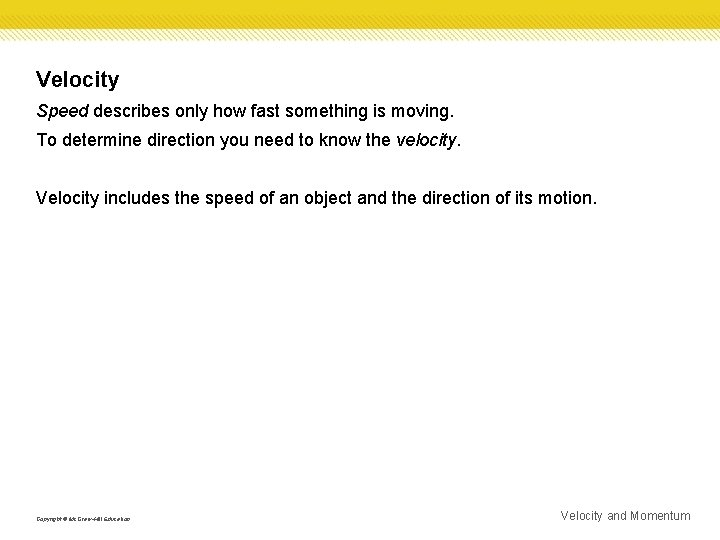Velocity Speed describes only how fast something is moving. To determine direction you need to know the velocity. Velocity includes the speed of an object and the direction of its motion. Copyright © Mc. Graw-Hill Education Velocity and MomentumRelative Motion If you are sitting in a chair reading this sentence, you are moving. You are not moving relative to your desk or your school building, but you are moving relative to the other planets in the solar system and the Sun. Copyright © Mc. Graw-Hill Education Velocity and MomentumMomentum A moving object has a property called momentum that is related to how much force is needed to change its motion The momentum of an object is the product of its mass and velocity. Copyright © Mc. Graw-Hill Education Velocity and Momentum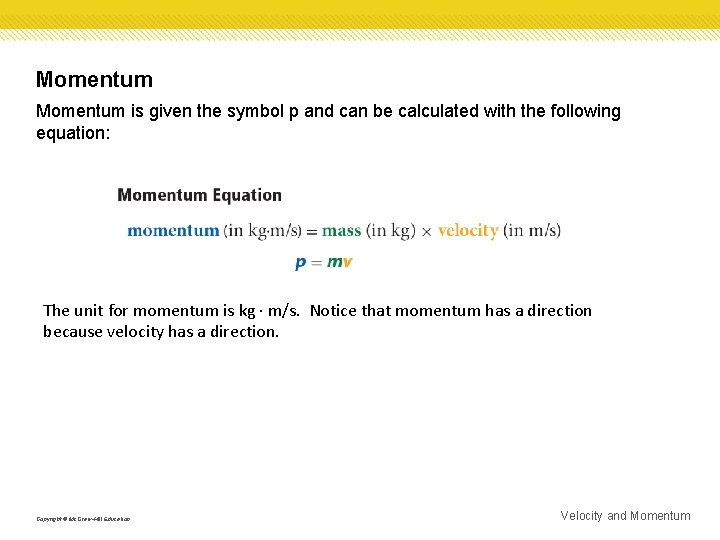Momentum is given the symbol p and can be calculated with the following equation: The unit for momentum is kg · m/s. Notice that momentum has a direction because velocity has a direction. Copyright © Mc. Graw-Hill Education Velocity and Momentum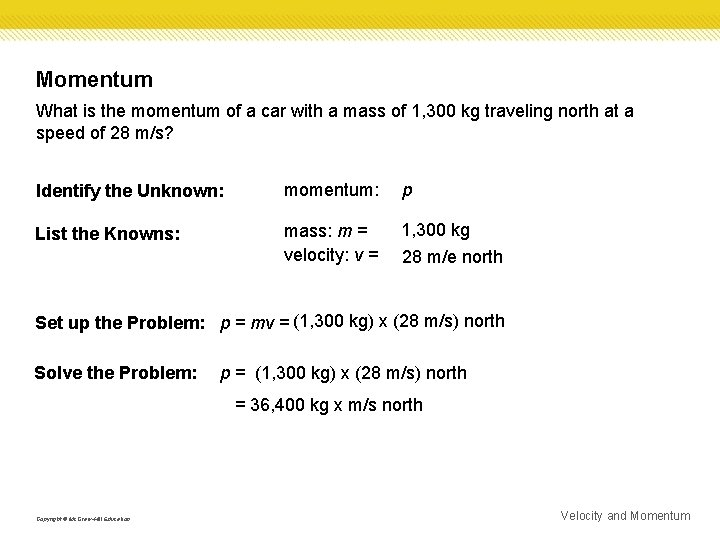Momentum What is the momentum of a car with a mass of 1, 300 kg traveling north at a speed of 28 m/s? Identify the Unknown: momentum: p List the Knowns: mass: m = velocity: v = 1, 300 kg 28 m/e north Set up the Problem: p = mv = (1, 300 kg) x (28 m/s) north Solve the Problem: p = (1, 300 kg) x (28 m/s) north = 36, 400 kg x m/s north Copyright © Mc. Graw-Hill Education Velocity and Momentum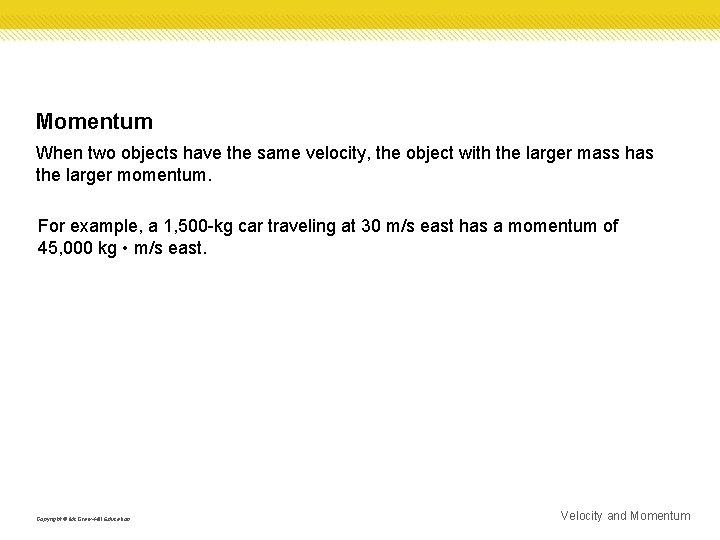Momentum When two objects have the same velocity, the object with the larger mass has the larger momentum. For example, a 1, 500 -kg car traveling at 30 m/s east has a momentum of 45, 000 kg • m/s east. Copyright © Mc. Graw-Hill Education Velocity and Momentum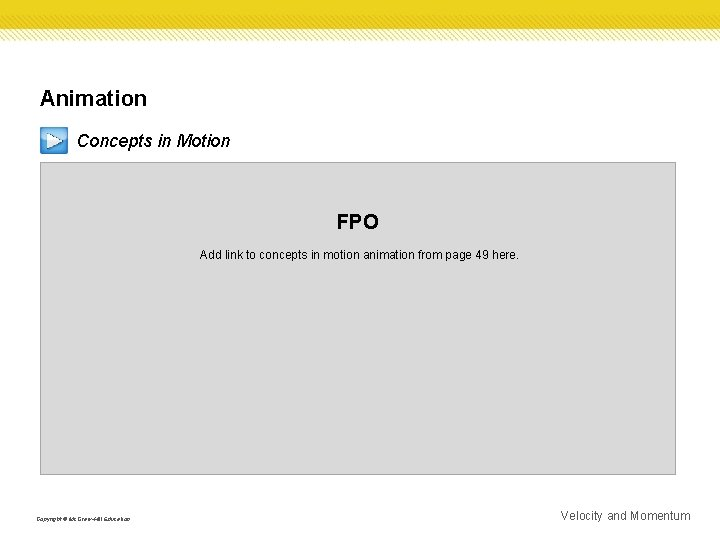Animation Concepts in Motion FPO Add link to concepts in motion animation from page 49 here. Copyright © Mc. Graw-Hill Education Velocity and Momentum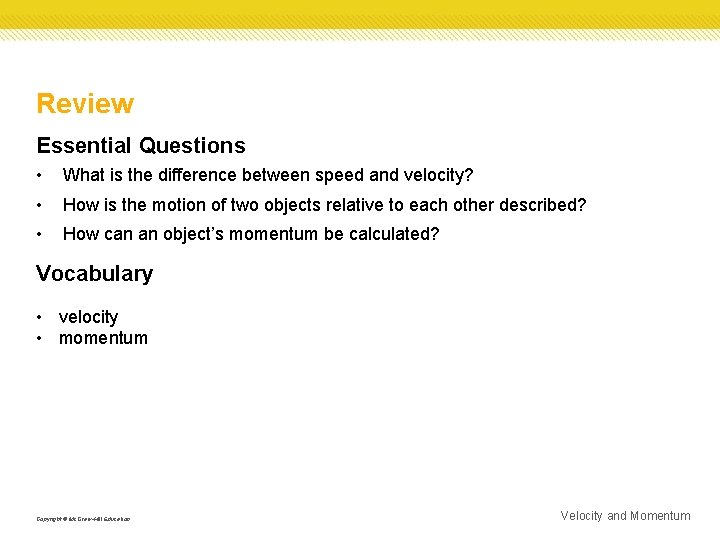Review Essential Questions • What is the difference between speed and velocity? • How is the motion of two objects relative to each other described? • How can an object’s momentum be calculated? Vocabulary • velocity • momentum Copyright © Mc. Graw-Hill Education Velocity and Momentum